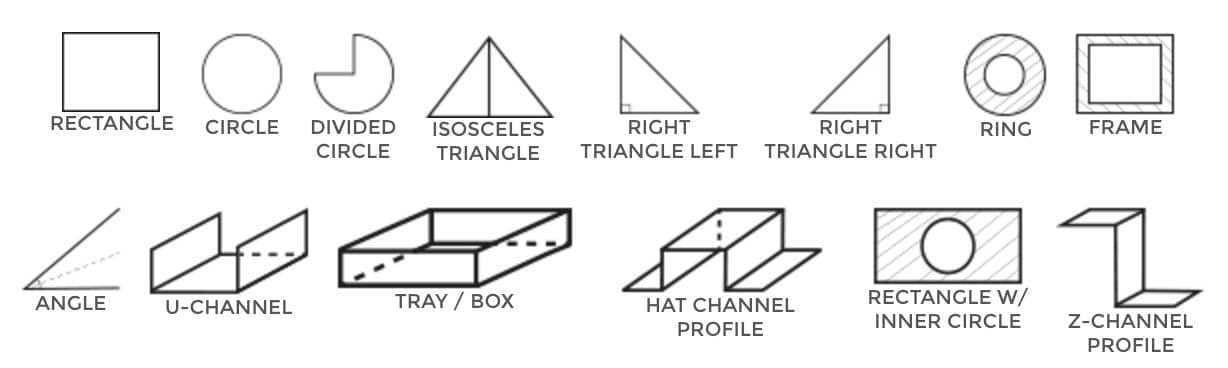# Let's talk shapes and forms you can order right on MetalsCut4U

Let's talk shapes and forms you can order right on MetalsCut4U

When talking about shapes and forms we mean all kind of geometrical forms that can be cut, bend and possibly welded for you
We have currently 14 different shapes you can order online on our website by entering your measurements defining the size of the shape you would like to have or need.A rectangle is a four sided flat shape where every angle is a right angle (90 degrees) In order to define a rectangle you need to 2 linear measures. The opposite side is 100% identical. Based on those two measures you can calculate the area and the range. A square is also a “sort” of rectangle. The difference to a rectangle is that all sides are of equal length.

A circle is a 2 dimensional shape where the rim / border is always the same distance from the center. The distance between the center and the circumference is called radius.The diameter is defined as 2 times the radius. Based on this radius we can calculate the area and the range a circle.

A partial/divided circle is a circular segment, that describes a region of a circle. A full circle has 360 degrees, a partial circle will always have less than 360 degrees.The difference between 360 and the circular segment you may want to have will be cut of.

Mathematically spoken a isosceles triangle is a triangle, where at least two sides have equal length and at least two angles are equal as well.In order to calculate the area and the range of a isosceles triangle you will be asked to enter the length of side (a or b) and the length of side c.

A right triangle always will have these 2 rules that describe a right triangle a) it has one right angle (which is always 90 degrees).b) it has two other equal angles witch always be 45 degrees.

A ring is basically a circle with an inner cut out, which is also a circle. For us to calculate a ring you will need to enter the outer radius and the inner radius of a circle.

The frame describes much like in the case of a ring an outer rim and a inner rim. The shape of a frame can be a rectangle or a square. You will need to give us the measures of side a and b for the outer rim and side c and d for the inner rim.

The angle is basically “L”-shaped. It can be very long for instance to protect a corner of a wall or it could be fairly short to hold something together. Whatever you will be using it for you define the by entering the measure of side a and b as well as the length. A typical L-shaped angle has a 90 degree corner, but with us your angle can have other degrees as well.

The U Channel (U-channel sometimes also called a C-Channel) is a metal rail where two sides are bend in an 90 degree angle to form a U. It is formed out of a rectangle where second step two sides are getting bend to shape a U. You can define your U-Channel as you wish. By entering the measures of side a, b and c and the length of the rail we can quote you a price.

The Tray is formed like a box. You define the inner rectangle and the height of the sides of the trat. Please keep in mind if you want the sides to be welded together you will need to choose the optional box when you order a tray or box .

The Hat Channel ( Hat-Channel ) is composed of a U Channel ( U-Channel ) with 2 outward flanges on each side. If you look at it from the side it has the form of a top hat.you will need to give us 5 measures to define the hat channel. Please be aware that the total length of those 5 measures can not exceed 48 inches.

The rectangle with an inner circle is exactly what it is. For us to cut you such a form for you will be asked to enter the outer dimensions of the rectangle and the radius of the circle. The circle radius can not exceed the the measure of side a or b and is always centered in the rectangle.

The Z-Channel ( Z channel ) also known as a Z-Bar, Zee or Purlin is metal shaped into a "Z". In our case the Z Channel has two 90 degree angles. You will need to define your Z-Channel by entering the measures of the sides a, b and c as well as the length you need. That is all to configure your individual custom cut sheet metal.

Sheet metal like aluminum, stainless or carbon steel are metals we can cut, bend and weld to your specs!

Sheet metal - we cut it - we bend it - we weld it - 4U.